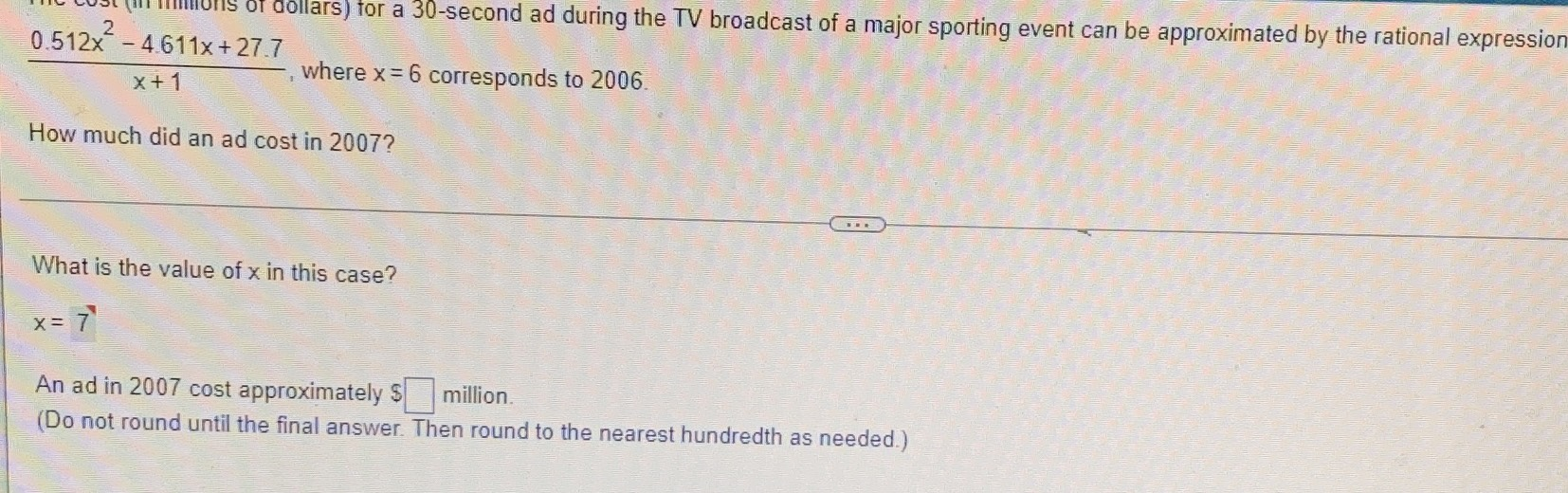### ¿Todavía tienes preguntas de matemáticas?

Pregunte a nuestros tutores expertos
Algebra
Pregunta$$\frac { 0.512 x ^ { 2 } - 4.611 x + 27.7 } { x + 1 }$$ , where $$x = 6$$ corresponds to $$2006$$ . How much did an ad cost in $$2007 ?$$

What is the value of $$x$$ in this case?

$$x = 7$$

An ad in $$2007$$ cost approximately $$\ \square$$ million.

(Do not round until the final answer. Then round to the nearest hundredth as needed.)